# k map 3

## Larger 4-variable Karnaugh maps

Knowing how to generate Gray code should allow us to build larger maps. Actually, all we need to do is look at the left to right sequence across the top

of the 3-variable map, and copy it down the left side of the 4-variable map. See below.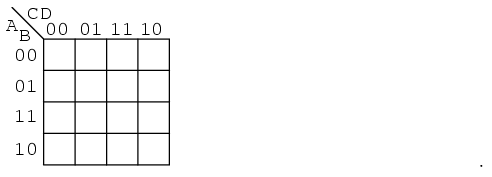The following four variable Karnaugh maps illustrate reduction of Boolean expressions too tedious for Boolean algebra. Reductions could be done with

Boolean algebra. However, the Karnaugh map is faster and easier, especially if there are many logic reductions to do.The above Boolean expression has seven product terms. They are mapped top to bottom and left to right on the K-map above. For example, the first P-term

A’B’CD is first row 3rd cell, corresponding to map location A=0, B=0, C=1, D=1. The other product terms are placed in a similar manner.

Encircling the largest groups possible, two groups of four are shown above. The dashed horizontal group corresponds the the simplified product term

AB. The vertical group corresponds to Boolean CD. Since there are two groups, there will be two product terms in the Sum-Of-Products result of

Out=AB+CD.

Fold up the corners of the map below like it is a napkin to make the four cells physically adjacent.The four cells above are a group of four because they all have the Boolean variablesB’ and D’ in common. In other words, B=0 for the

four cells, and D=0 for the four cells. The other variables (A, B) are 0 in some cases, 1 in other cases with respect to the

four corner cells. Thus, these variables (A, B) are not involved with this group of four. This single group comes out of the map as one product term

for the simplified result: Out=B’C’

For the K-map below, roll the top and bottom edges into a cylinder forming eight adjacent cells.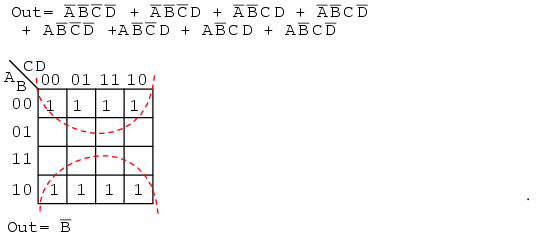The above group of eight has one Boolean variable in common: B=0. Therefore, the one group of eight is covered by one p-term: B’. The original

eight term Boolean expression simplifies to Out=B’

The Boolean expression below has nine p-terms, three of which have three Booleans instead of four. The difference is that while four Boolean variable

product terms cover one cell, the three Boolean p-terms cover a pair of cells each.The six product terms of four Boolean variables map in the usual manner above as single cells. The three Boolean variable terms (three each) map as cell

pairs, which is shown above. Note that we are mapping p-terms into the K-map, not pulling them out at this point.

For the simplification, we form two groups of eight. Cells in the corners are shared with both groups. This is fine. In fact, this leads to a better

solution than forming a group of eight and a group of four without sharing any cells. Final Solution is Out=B’+D’

Below we map the unsimplified Boolean expression to the Karnaugh map.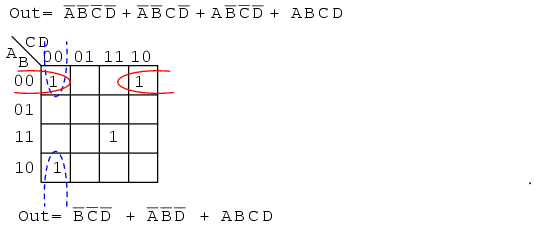Above, three of the cells form into a groups of two cells. A fourth cell cannot be combined with anything, which often happens in “real world” problems. In

this case, the Boolean p-term ABCD is unchanged in the simplification process. Result: Out= B’C’D’+A’B’D’+ABCD

Often times there is more than one minimum cost solution to a simplification problem. Such is the case illustrated below.Both results above have four product terms of three Boolean variable each. Both are equally valid minimal cost solutions. The difference in the final

solution is due to how the cells are grouped as shown above. A minimal cost solution is a valid logic design with the minimum number of gates with the

minimum number of inputs.

Below we map the unsimplified Boolean equation as usual and form a group of four as a first simplification step. It may not be obvious how to pick up the

remaining cells.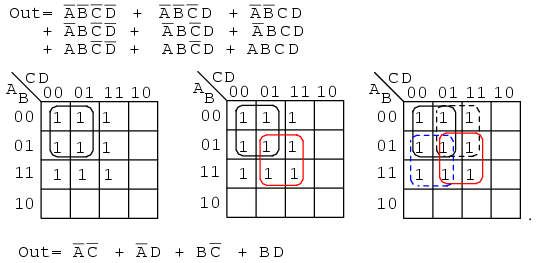Pick up three more cells in a group of four, center above. There are still two cells remaining. the minimal cost method to pick up those is to group them

with neighboring cells as groups of four as at above right.

On a cautionary note, do not attempt to form groups of three. Groupings must be powers of 2, that is, 1, 2, 4, 8 …

Below we have another example of two possible minimal cost solutions. Start by forming a couple of groups of four after mapping the cells.The two solutions depend on whether the single remaining cell is grouped with the first or the second group of four as a group of two cells. That cell

either comes out as either ABC’ or ABD, your choice. Either way, this cell is covered by either Boolean product term. Final results are shown

above.

Below we have an example of a simplification using the Karnaugh map at left or Boolean algebra at right. Plot C’/b> on the map as the area of all cells

covered by address C=0, the 8-cells on the left of the map. Then, plot the single ABCD cell. That single cell forms a group of 2-cell as

shown, which simplifies to P-term ABD, for an end result of Out = C’ + ABD.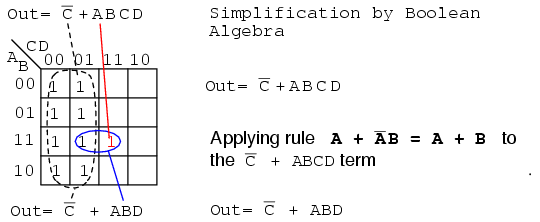This (above) is a rare example of a four variable problem that can be reduced with Boolean algebra without a lot of work, assuming that you remember the

theorems.

## Minterm vs maxterm solution

So far we have been finding Sum-Of-Product (SOP) solutions to logic reduction problems. For each of these SOP solutions, there is also a Product-Of-Sums

solution (POS), which could be more useful, depending on the application. Before working a Product-Of-Sums solution, we need to introduce some new

terminology. The procedure below for mapping product terms is not new to this chapter. We just want to establish a formal procedure for minterms for

comparison to the new procedure for maxterms.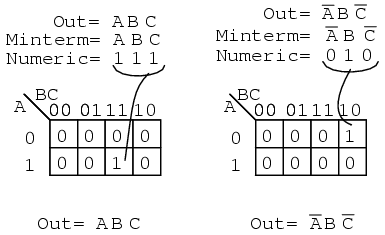A minterm is a Boolean expression resulting in 1 for the output of a single cell, and 0s for all other cells in a Karnaugh map, or

truth table. If a minterm has a single 1 and the remaining cells as 0s, it would appear to cover a minimum area of 1s. The illustration

above left shows the minterm ABC, a single product term, as a single 1 in a map that is otherwise 0s. We have not shown the 0s

in our Karnaugh maps up to this point, as it is customary to omit them unless specifically needed. Another minterm A’BC’ is shown above right. The

point to review is that the address of the cell corresponds directly to the minterm being mapped. That is, the cell 111 corresponds to the minterm

ABC above left. Above right we see that the minterm A’BC’ corresponds directly to the cell 010. A Boolean expression or map may have

multiple minterms.

Referring to the above figure, Let’s summarize the procedure for placing a minterm in a K-map:

Identify the minterm (product term) term to be mapped.
Write the corresponding binary numeric value.
Use binary value as an address to place a 1 in the K-map
Repeat steps for other minterms (P-terms within a Sum-Of-Products).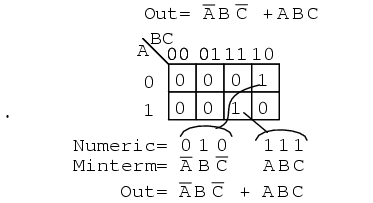A Boolean expression will more often than not consist of multiple minterms corresponding to multiple cells in a Karnaugh map as shown above. The multiple

minterms in this map are the individual minterms which we examined in the previous figure above. The point we review for reference is that the 1s

come out of the K-map as a binary cell address which converts directly to one or more product terms. By directly we mean that a 0 corresponds to a

complemented variable, and a 1 corresponds to a true variable. Example: 010 converts directly to A’BC’. There was no reduction in this

example. Though, we do have a Sum-Of-Products result from the minterms.

Referring to the above figure, Let’s summarize the procedure for writing the Sum-Of-Products reduced Boolean equation from a K-map:

Form largest groups of 1s possible covering all minterms. Groups must be a power of 2.
Write binary numeric value for groups.
Convert binary value to a product term.
Repeat steps for other groups. Each group yields a p-terms within a Sum-Of-Products.

Nothing new so far, a formal procedure has been written down for dealing with minterms. This serves as a pattern for dealing with maxterms.

Next we attack the Boolean function which is 0 for a single cell and 1s for all others.A maxterm is a Boolean expression resultingin a 0 for the output of a single cell expression, and 1s for all other cells in the

Karnaugh map, or truth table. The illustration above left shows the maxterm (A+B+C), a single sum term, as a single 0 in a map that is

otherwise 1s. If a maxterm has a single 0 and the remaining cells as 1s, it would appear to cover a maximum area of 1s.

There are some differences now that we are dealing with something new, maxterms. The maxterm is a 0, not a 1 in the Karnaugh map. A maxterm

is a sum term, (A+B+C) in our example, not a product term.

It also looks strange that (A+B+C) is mapped into the cell 000. For the equation Out=(A+B+C)=0, all three variables (A, B, C)

must individually be equal to 0. Only (0+0+0)=0 will equal 0. Thus we place our sole 0 for minterm (A+B+C) in cell

A,B,C=000 in the K-map, where the inputs are all0 . This is the only case which will give us a 0 for our maxterm. All other cells

contain 1s because any input values other than ((0,0,0) for (A+B+C) yields 1s upon evaluation.

Referring to the above figure, the procedure for placing a maxterm in the K-map is:

Identify the Sum term to be mapped.
Write corresponding binary numeric value.
Form the complement
Use the complement as an address to place a 0 in the K-map
Repeat for other maxterms (Sum terms within Product-of-Sums expression).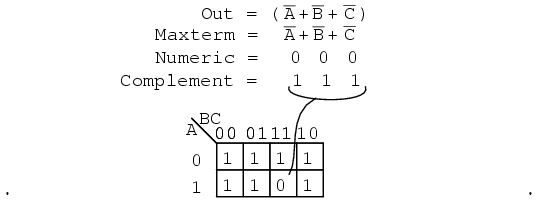Another maxterm A’+B’+C’ is shown above. Numeric 000 corresponds to A’+B’+C’. The complement is 111. Place a 0 for

maxterm (A’+B’+C’) in this cell (1,1,1) of the K-map as shown above.

Why should (A’+B’+C’) cause a 0 to be in cell 111? When A’+B’+C’ is (1’+1’+1′), all 1s in, which is

(0+0+0) after taking complements, we have the only condition that will give us a 0. All the 1s are complemented to all 0s, which

is 0 when ORed.A Boolean Product-Of-Sums expression or map may have multiple maxterms as shown above. Maxterm (A+B+C) yields numeric 111 which complements

to 000, placing a 0 in cell (0,0,0). Maxterm (A+B+C’) yields numeric 110 which complements to 001, placing a

0 in cell (0,0,1).

Now that we have the k-map setup, what we are really interested in is showing how to write a Product-Of-Sums reduction. Form the 0s into groups.

That would be a group of two below. Write the binary value corresponding to the sum-term which is (0,0,X). Both A and B are 0 for the group.

But, C is both 0 and 1 so we write an X as a place holder for C. Form the complement (1,1,X). Write the Sum-term

(A+B) discarding the C and the X which held its’ place. In general, expect to have more sum-terms multiplied together in the

Product-Of-Sums result. Though, we have a simple example here.Let’s summarize the procedure for writing the Product-Of-Sums Boolean reduction for a K-map:

Form largest groups of 0s possible, covering all maxterms. Groups must be a power of 2.
Write binary numeric value for group.
Complement binary numeric value for group.
Convert complement value to a sum-term.
Repeat steps for other groups. Each group yields a sum-term within a Product-Of-Sums result.

Example:

Simplify the Product-Of-Sums Boolean expression below, providing a result in POS form.Solution:

Transfer the seven maxterms to the map below as 0s. Be sure to complement the input variables in finding the proper cell location.We map the 0s as they appear left to right top to bottom on the map above. We locate the last three maxterms with leader lines..

Once the cells are in place above, form groups of cells as shown below. Larger groups will give a sum-term with fewer inputs. Fewer groups will yield fewer

sum-terms in the result.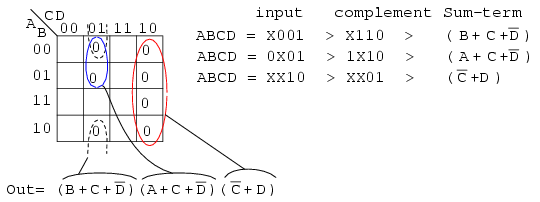We have three groups, so we expect to have three sum-terms in our POS result above. The group of 4-cells yields a 2-variable sum-term. The two groups of

2-cells give us two 3-variable sum-terms. Details are shown for how we arrived at the Sum-terms above. For a group, write the binary group input address,

then complement it, converting that to the Boolean sum-term. The final result is product of the three sums.

Example:

Simplify the Product-Of-Sums Boolean expression below, providing a result in SOP form.Solution:

This looks like a repeat of the last problem. It is except that we ask for a Sum-Of-Products Solution instead of the Product-Of-Sums which we just

finished. Map the maxterm 0s from the Product-Of-Sums given as in the previous problem, below left.Then fill in the implied 1s in the remaining cells of the map above right.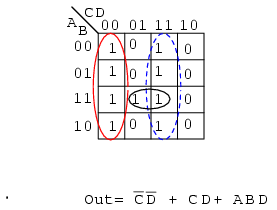Form groups of 1s to cover all 1s. Then write the Sum-Of-Products simplified result as in the previous section of this chapter. This is

identical to a previous problem.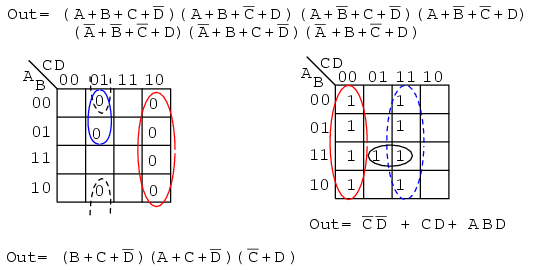Above we show both the Product-Of-Sums solution, from the previous example, and the Sum-Of-Products solution from the current problem for comparison. Which

is the simpler solution? The POS uses 3-OR gates and 1-AND gate, while the SOP uses 3-AND gates and 1-OR gate. Both use four gates each. Taking a closer

look, we count the number of gate inputs. The POS uses 8-inputs; the SOP uses 7-inputs. By the definition of minimal cost solution, the SOP solution is

simpler. This is an example of a technically correct answer that is of little use in the real world.

The better solution depends on complexity and the logic family being used. The SOP solution is usually better if using the TTL logic family, as NAND gates

are the basic building block, which works well with SOP implementations. On the other hand, A POS solution would be acceptable when using the CMOS logic

family since all sizes of NOR gates are available.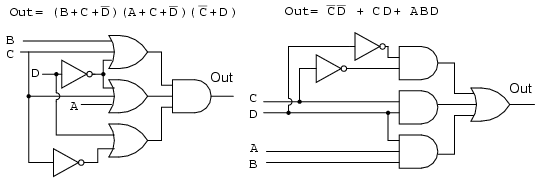The gate diagrams for both cases are shown above, Product-Of-Sums left, and Sum-Of-Products right.

Below, we take a closer look at the Sum-Of-Products version of our example logic, which is repeated at left.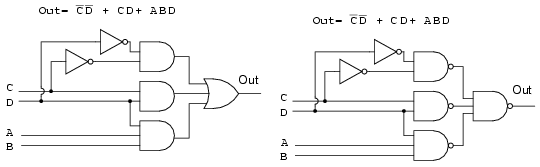Above all AND gates at left have been replaced by NAND gates at right.. The OR gate at the output is replaced by a NAND gate. To prove that AND-OR logic

is equivalent to NAND-NAND logic, move the inverter invert bubbles at the output of the 3-NAND gates to the input of the final NAND as shown in going from

above right to below left.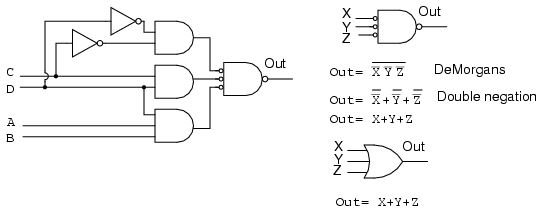Above right we see that the output NAND gate with inverted inputs is logically equivalent to an OR gate by DeMorgan’s theorem and double negation. This

information is useful in building digital logic in a lboratory setting where TTL logic family NAND gates are more readily available in a wide variety of

configurations than other types.

The Procedure for constructing NAND-NAND logic, in place of AND-OR logic is as follows:

Produce a reduced Sum-Of-Products logic design.
When drawing the wiring diagram of the SOP, replace all gates (both AND and OR) with NAND gates.
Unused inputs should be tied to logic High.
In case of troubleshooting, internal nodes at the first level of NAND gate outputs do NOT match AND-OR diagram logic levels, but are inverted. Use the

NAND-NAND logic diagram. Inputs and final output are identical, though.
Label any multiple packages U1, U2,.. etc.
Use data sheet to assign pin numbers to inputs and outputs of all gates.

Example:

Let us revisit a previous problem involving an SOP minimization. Produce a Product-Of-Sums solution. Compare the POS solution to the previous SOP.Solution:

Above left we have the original problem starting with a 9-minterm Boolean unsimplified expression. Reviewing, we formed four groups of 4-cells to yield a

4-product-term SOP result, lower left.

In the middle figure, above, we fill in the empty spaces with the implied 0s. The 0s form two groups of 4-cells. The solid red group is

(A’+B), the dashed red group is (C’+D). This yields two sum-terms in the Product-Of-Sums result, above right Out = (A’+B)(C’+D)

Comparing the previous SOP simplification, left, to the POS simplification, right, shows that the POS is the least cost solution. The SOP uses 5-gates

total, the POS uses only 3-gates. This POS solution even looks attractive when using TTL logic due to simplicity of the result. We can find AND gates and an

OR gate with 2-inputs.The SOP and POS gate diagrams are shown above for our comparison problem.

Given the pin-outs for the TTL logic family integrated circuit gates below, label the maxterm diagram above right with Circuit designators (U1-a, U1-b,

U2-a, etc), and pin numbers.Each integrated circuit package that we use will receive a circuit designator: U1, U2, U3. To distinguish between the individual gates within the package,

they are identified as a, b, c, d, etc. The 7404 hex-inverter package is U1. The individual inverters in it are are U1-a, U1-b, U1-c, etc. U2 is assigned to

the 7432 quad OR gate. U3 is assigned to the 7408 quad AND gate. With reference to the pin numbers on the package diagram above, we assign pin numbers to

all gate inputs and outputs on the schematic diagram below.

We can now build this circuit in a laboratory setting. Or, we could design a printed circuit board for it. A printed circuit board contains copper

foil “wiring” backed by a non conductive substrate of phenolic, or epoxy-fiberglass. Printed circuit boards are used to mass produce electronic circuits.

Ground the inputs of unused gates.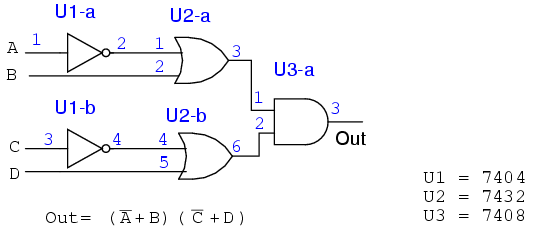Label the previous POS solution diagram above left (third figure back) with Circuit designators and pin numbers. This will be similar to what we just did.We can find 2-input AND gates, 7408 in the previous example. However, we have trouble finding a 4-input OR gate in our TTL catalog. The only kind of gate

with 4-inputs is the 7420 NAND gate shown above right.

We can make the 4-input NAND gate into a 4-input OR gate by inverting the inputs to the NAND gate as shown below. So we will use the 7420 4-input NAND gate

as an OR gate by inverting the inputs.The result is shown above. It is the only practical way to actually build it with TTL gates by using NAND-NAND logic replacing AND-OR logic.

## Σ (sum) and Π (product) notation

For reference, this section introduces the terminology used in some texts to describe the minterms and maxterms assigned to a Karnaugh map. Otherwise, there

is no new material here.

Σ (sigma) indicates sum and lower case “m” indicates minterms. Σm indicates sum of minterms. The following example is revisited to illustrate our

point. Instead of a Boolean equation description of unsimplified logic, we list the minterms.

f(A,B,C,D) = Σ m(1, 2, 3, 4, 5, 7, 8, 9, 11, 12, 13, 15)

or

f(A,B,C,D) =

Σ(m1,m2,m3,m4,m5,m7,m8,m9,m11,m12,m1

3,m15)

The numbers indicate cell location, or address, within a Karnaugh map as shown below right. This is certainly a compact means of describing a list of

minterms or cells in a K-map.The Sum-Of-Products solution is not affected by the new terminology. The minterms, 1s, in the map have been grouped as usual and a Sum-OF-Products

solution written.

Below, we show the terminology for describing a list of maxterms. Product is indicated by the Greek Π (pi), and upper case “M” indicates maxterms.

ΠM indicates product of maxterms. The same example illustrates our point. The Boolean equation description of unsimplified logic, is replaced by a list

of maxterms.

f(A,B,C,D) = Π M(2, 6, 8, 9, 10, 11, 14)

or

f(A,B,C,D) = Π(M2, M6, M8, M9, M10, M11, M14)

Once again, the numbers indicate K-map cell address locations. For maxterms this is the location of 0s, as shown below. A Product-OF-Sums solution is

completed in the usual manner.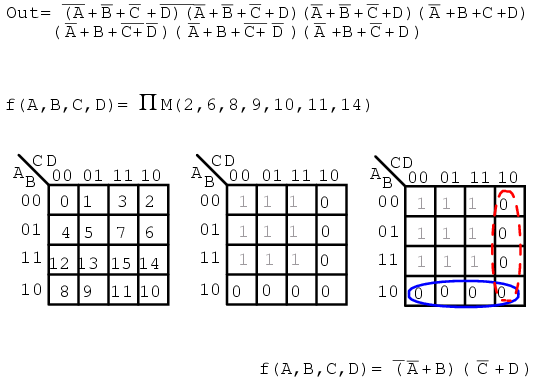## Don’t care cells in the Karnaugh map

Up to this point we have considered logic reduction problems where the input conditions were completely specified. That is, a 3-variable truth table or

Karnaugh map had 2n = 23 or 8-entries, a full table or map. It is not always necessary to fill in the complete truth table for some

real-world problems. We may have a choice to not fill in the complete table.

For example, when dealing with BCD (Binary Coded Decimal) numbers encoded as four bits, we may not care about any codes above the BCD range of (0, 1,

2…9). The 4-bit binary codes for the hexadecimal numbers (Ah, Bh, Ch, Eh, Fh) are not valid BCD codes. Thus, we do not have to fill in those codes at

the end of a truth table, or K-map, if we do not care to. We would not normally care to fill in those codes because those codes (1010, 1011, 1100, 1101,

1110, 1111) will never exist as long as we are dealing only with BCD encoded numbers. These six invalid codes are don’t cares as far as we are

concerned. That is, we do not care what output our logic circuit produces for these don’t cares.

Don’t cares in a Karnaugh map, or truth table, may be either 1s or 0s, as long as we don’t care what the output is for an input condition we

never expect to see. We plot these cells with an asterisk, *, among the normal 1s and b>0s. When forming groups of cells, treat the don’t care

cell as either a 1 or a 0, or ignore the don’t cares. This is helpful if it allows us to form a larger group than would otherwise be possible

without the don’t cares. There is no requirement to group all or any of the don’t cares. Only use them in a group if it simplifies the logic.Above is an example of a logic function where the desired output is 1 for input ABC = 101 over the range from 000 to 101. We do not

care what the output is for the other possible inputs (110, 111). Map those two as don’t cares. We show two solutions. The solution on the right Out

= AB’C is the more complex solution since we did not use the don’t care cells. The solution in the middle, Out=AC, is less complex because we grouped a

don’t care cell with the single 1 to form a group of two. The third solution, a Product-Of-Sums on the right, results from grouping a don’t care

with three zeros forming a group of four 0s. This is the same, less complex, Out=AC. We have illustrated that the don’t care cells may be

used as either 1s or 0s, whichever is useful.The electronics class of Lightning State College has been asked to build the lamp logic for a stationary bicycle exhibit at the local science museum. As a

rider increases his pedaling speed, lamps will light on a bar graph display. No lamps will light for no motion. As speed increases, the lower lamp, L1

lights, then L1 and L2, then, L1, L2, and L3, until all lamps light at the highest speed. Once all the lamps illuminate, no further increase in speed will

have any effect on the display.

A small DC generator coupled to the bicycle tire outputs a voltage proportional to speed. It drives a tachometer board which limits the voltage at the high

end of speed where all lamps light. No further increase in speed can increase the voltage beyond this level. This is crucial because the downstream A to D

(Analog to Digital) converter puts out a 3-bit code, ABC, 23 or 8-codes, but we only have five lamps. A is the most significant

bit, C the least significant bit.

The lamp logic needs to respond to the six codes out of the A to D. For ABC=000, no motion, no lamps light. For the five codes (001 to 101)

lamps L1, L1&L2, L1&L2&L3, up to all lamps will light, as speed, voltage, and the A to D code (ABC) increases. We do not care about the

response to input codes (110, 111) because these codes will never come out of the A to D due to the limiting in the tachometer block. We need to

design five logic circuits to drive the five lamps.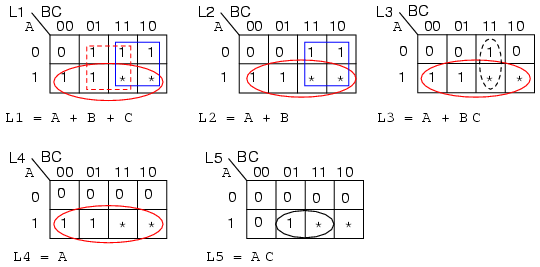Since, none of the lamps light for ABC=000 out of the A to D, enter a 0 in all K-maps for cell ABC=000. Since we don’t care about the

never to be encountered codes (110, 111), enter asterisks into those two cells in all five K-maps.

Lamp L5 will only light for code ABC=101. Enter a 1 in that cell and five 0s into the remaining empty cells of L5 K-map.

L4 will light initially for code ABC=100, and will remain illuminated for any code greater, ABC=101, because all lamps below L5 will light

when L5 lights. Enter 1s into cells 100 and 101 of the L4 map so that it will light for those codes. Four 0‘s fill the remaining

L4 cells

L3 will initially light for code ABC=011. It will also light whenever L5 and L4 illuminate. Enter three 1s into cells 011, 100, 101 for

L3 map. Fill three 0s into the remaining L3 cells.

L2 lights for ABC=010 and codes greater. Fill 1s into cells 010,011, 100, 101, and two 0s in the remaining cells.

The only time L1 is not lighted is for no motion. There is already a 0 in cell ABC=000. All the other five cells receive 1s.

Group the 1‘s as shown above, using don’t cares whenever a larger group results. The L1 map shows three product terms, corresponding to three groups

of 4-cells. We used both don’t cares in two of the groups and one don’t care on the third group. The don’t cares allowed us to form groups of four.

In a similar manner, the L2 and L4 maps both produce groups of 4-cells with the aid of the don’t care cells. The L4 reduction is striking in that the L4

lamp is controlled by the most significant bit from the A to D converter, L5=A. No logic gates are required for lamp L4. In the L3 and L5 maps,

single cells form groups of two with don’t care cells. In all five maps, the reduced Boolean equation is less complex than without the don’t cares.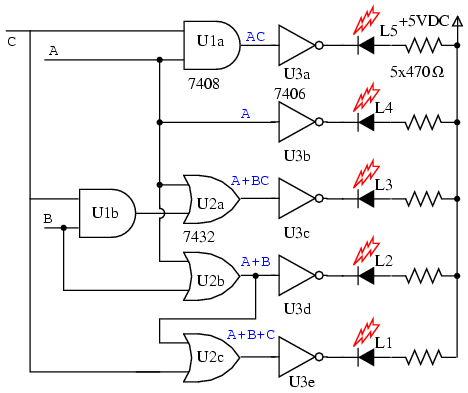The gate diagram for the circuit is above. The outputs of the five K-map equations drive inverters. Note that the L1 OR gate is not a 3-input gate

but a 2-input gate having inputs (A+B), C, outputting A+B+C The open collector inverters, 7406, are desirable for driving LEDs,

though, not part of the K-map logic design. The output of an open collecter gate or inverter is open circuited at the collector internal to the integrated

circuit package so that all collector current may flow through an external load. An active high into any of the inverters pulls the output low, drawing

current through the LED and the current limiting resistor. The LEDs would likely be part of a solid state relay driving 120VAC lamps for a museum exhibit,

not shown here.

## Larger 5 & 6-variable Karnaugh maps

Larger Karnaugh maps reduce larger logic designs. How large is large enough? That depends on the number of inputs, fan-ins, to the logic circuit

under consideration. One of the large programmable logic companies has an answer.

Altera’s own data, extracted from its library of customer designs, supports the value of heterogeneity. By examining logic cones, mapping them onto

LUT-based nodes and sorting them by the number of inputs that would be best at each node, Altera found that the distribution of fan-ins was nearly flat

between two and six inputs, with a nice peak at five.

The answer is no more than six inputs for most all designs, and five inputs for the average logic design. The five variable Karnaugh map follows.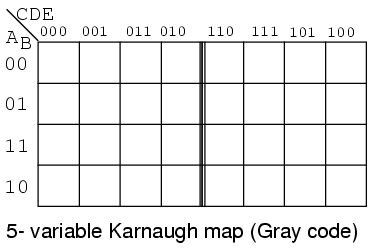The older version of the five variable K-map, a Gray Code map or reflection map, is shown above. The top (and side for a 6-variable map) of the map is

numbered in full Gray code. The Gray code reflects about the middle of the code. This style map is found in older texts. The newer preferred style is below.The overlay version of the Karnaugh map, shown above, is simply two (four for a 6-variable map) identical maps except for the most significant bit of the

3-bit address across the top. If we look at the top of the map, we will see that the numbering is different from the previous Gray code map. If we ignore

the most significant digit of the 3-digit numbers, the sequence 00, 01, 11, 10 is at the heading of both sub maps of the overlay map. The sequence of

eight 3-digit numbers is not Gray code. Though the sequence of four of the least significant two bits is.

Let’s put our 5-variable Karnaugh Map to use. Design a circuit which has a 5-bit binary input (A, B, C, D, E), with A being the MSB (Most Significant Bit).

It must produce an output logic High for any prime number detected in the input data.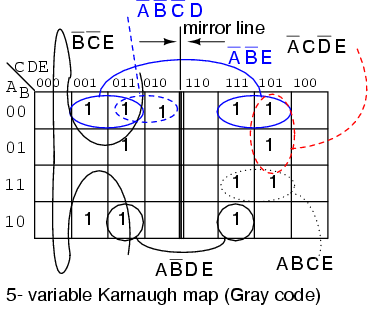We show the solution above on the older Gray code (reflection) map for reference. The prime numbers are (1,2,3,5,7,11,13,17,19,23,29,31). Plot a 1 in

each corresponding cell. Then, proceed with grouping of the cells. Finish by writing the simplified result. Note that 4-cell group A’B’E consists of two

pairs of cell on both sides of the mirror line. The same is true of the 2-cell group AB’DE. It is a group of 2-cells by being reflected about the mirror

line. When using this version of the K-map look for mirror images in the other half of the map.

Out = A’B’E + B’C’E + A’C’DE + A’CD’E + ABCE + AB’DE + A’B’C’D

Below we show the more common version of the 5-variable map, the overlay map.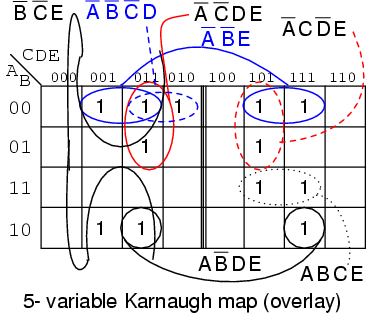If we compare the patterns in the two maps, some of the cells in the right half of the map are moved around since the addressing across the top of the map

is different. We also need to take a different approach at spotting commonality between the two halves of the map. Overlay one half of the map atop the

other half. Any overlap from the top map to the lower map is a potential group. The figure below shows that group AB’DE is composed of two stacked cells.

Group A’B’E consists of two stacked pairs of cells.

For the A’B’E group of 4-cells ABCDE = 00xx1 for the group. That is A,B,E are the same 001 respectively for the group. And,

CD=xx that is it varies, no commonality in CD=xx for the group of 4-cells. Since ABCDE = 00xx1, the group of 4-cells is covered by

A’B’XXE = A’B’E.The above 5-variable overlay map is shown stacked.

An example of a six variable Karnaugh map follows. We have mentally stacked the four sub maps to see the group of 4-cells corresponding to Out = C’F’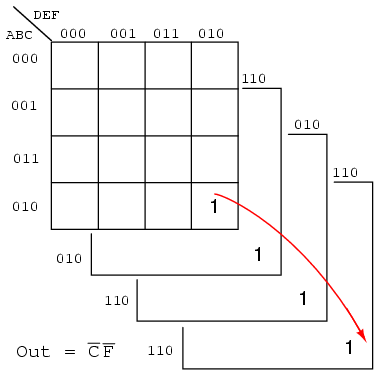A magnitude comparator (used to illustrate a 6-variable K-map) compares two binary numbers, indicating if they are equal, greater than, or less than each

other on three respective outputs. A three bit magnitude comparator has two inputs A2A1A0 and

B2B1B0
An integrated circuit magnitude comparator (7485) would actually have four inputs, But, the Karnaugh map below needs to be kept to a reasonable size. We

will only solve for the A>B output.

Below, a 6-variable Karnaugh map aids simplification of the logic for a 3-bit magnitude comparator. This is an overlay type of map. The binary address code

across the top and down the left side of the map is not a full 3-bit Gray code. Though the 2-bit address codes of the four sub maps is Gray code. Find

redundant expressions by stacking the four sub maps atop one another (shown above). There could be cells common to all four maps, though not in the example

below. It does have cells common to pairs of sub maps.The A>B output above is ABC>XYZ on the map below.Where ever ABC is greater than XYZ, a 1 is plotted. In the first line ABC=000 cannot be greater than any of the values of

XYZ. No 1s in this line. In the second line, ABC=001, only the first cell ABCXYZ= 001000 is ABC greater than XYZ.

A single 1 is entered in the first cell of the second line. The fourth line, ABC=010, has a pair of 1s. The third line, ABC=011

has three 1s. Thus, the map is filled with 1s in any cells where ABC is greater than XXZ.

In grouping cells, form groups with adjacentsub maps if possible. All but one group of 16-cells involves cells from pairs of the sub maps. Look for the

following groups:

1 group of 16-cells
2 groups of 8-cells
4 groups of 4-cells

The group of 16-cells, AX’ occupies all of the lower right sub map; though, we don’t circle it on the figure above.

One group of 8-cells is composed of a group of 4-cells in the upper sub map overlaying a similar group in the lower left map.
The second group of 8-cells is composed of a similar group of 4-cells in the right sub map overlaying the same group of 4-cells in the lower left map.

The four groups of 4-cells are shown on the Karnaugh map above with the associated product terms. Along with the product terms for the two groups of 8-cells

and the group of 16-cells, the final Sum-Of-Products reduction is shown, all seven terms. Counting the 1s in the map, there is a total of 16+6+6=28

ones. Before the K-map logic reduction there would have been 28 product terms in our SOP output, each with 6-inputs. The Karnaugh map yielded seven product

terms of four or less inputs. This is really what Karnaugh maps are all about!

The wiring diagram is not shown. However, here is the parts list for the 3-bit magnitude comparator for ABC>XYZ using 4 TTL logic family parts:

1 ea 7410 triple 3-input NAND gate AX’, ABY’, BX’Y’
2 ea 7420 dual 4-input NAND gate ABCZ’, ACY’Z’, BCX’Z’, CX’Y’Z’
1 ea 7430 8-input NAND gate for output of 7-P-terms

REVIEW:

Boolean algebra, Karnaugh maps, and CAD (Computer Aided Design) are methods of logic simplification. The goal of logic simplification is a minimal cost

solution.

A minimal cost solution is a valid logic reduction with the minimum number of gates with the minimum number of inputs.

Venn diagrams allow us to visualize Boolean expressions, easing the transition to Karnaugh maps.

Karnaugh map cells are organized in Gray code order so that we may visualize redundancy in Boolean expressions which results in simplification.

The more common Sum-Of-Products (Sum of Minters) expressions are implemented as AND gates (products) feeding a single OR gate (sum).

Sum-Of-Products expressions (AND-OR logic) are equivalent to a NAND-NAND implementation. All AND gates and OR gates are replaced by NAND gates.

Less often used, Product-Of-Sums expressions are implemented as OR gates (sums) feeding into a single AND gate (product). Product-Of-Sums expressions are

based on the 0s, maxterms, in a Karnaugh map.

### Related Posts:

COMMENT songs

CONTACT
FAVORITE
SITEMAP
VLSI COMPANIES IN BANGALORE
VLSI INTERVIEW QUESTION RFSIR.COM